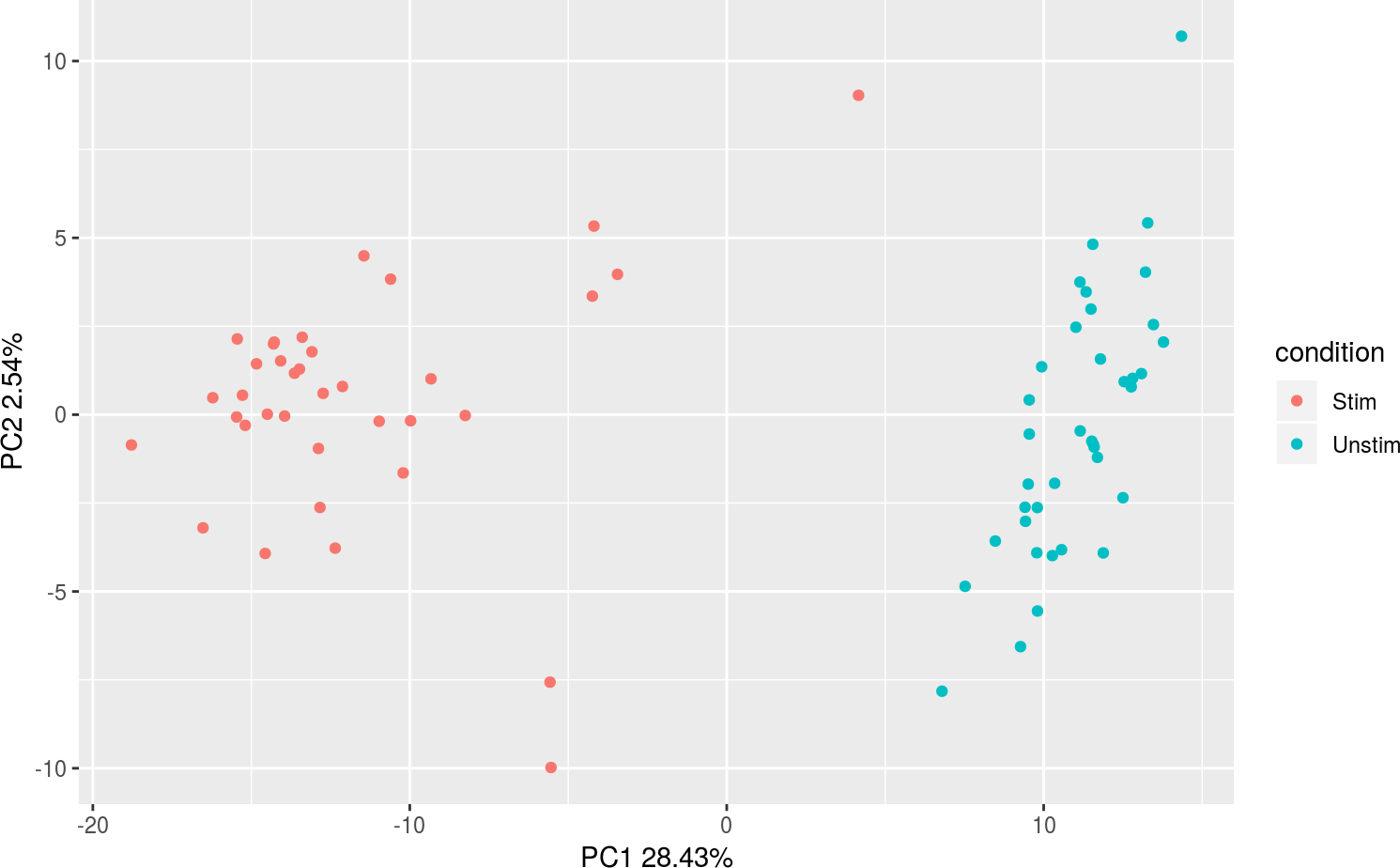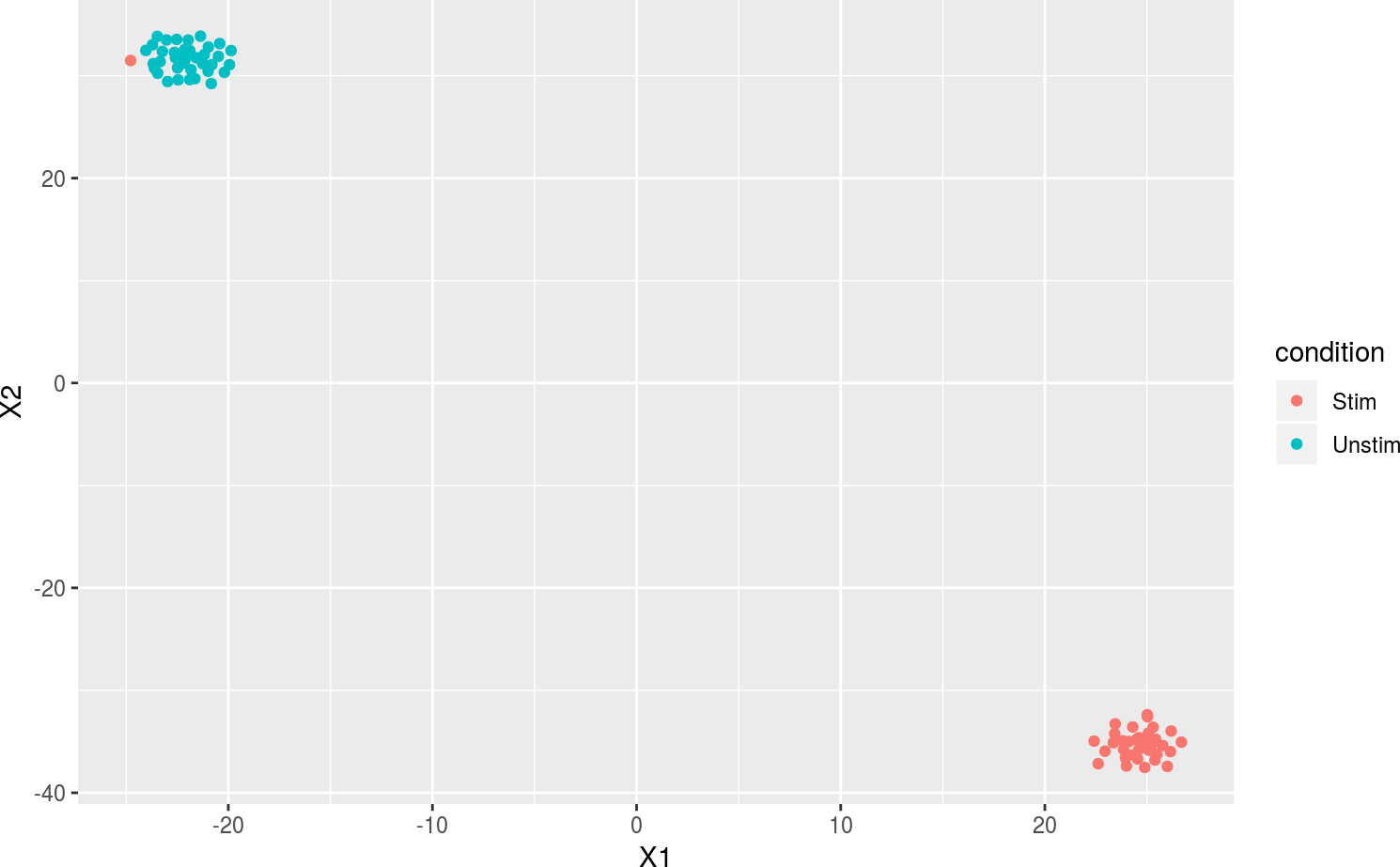# 1 Introduction

The analysis modules available through the Shiny app are also available as R functions for standard R console processing of single cell RNA-Seq data using a SCtkExperiment object. At any stage, you can load the Shiny App to interactively visualize and analyze a data set, but this vignette will show a standard workflow run entirely through the R console.

# 2 MAITS Example

The MAST package contains a convenient scRNA-Seq example data set of 96 Mucosal Associated Invariant T cells (MAITs), half of which were stimulated with cytokines to induce a response. For more details, consult the MAST package and vignette.

We will first convert the MAST example dataset to a SCtkExperiment object.

``````suppressPackageStartupMessages({
library(MAST)
library(singleCellTK)
library(xtable)
})

data(maits, package="MAST")
maits_sce <- createSCE(assayFile = t(maits\$expressionmat),
annotFile = maits\$cdat,
featureFile = maits\$fdat,
assayName = "logtpm",
inputDataFrames = TRUE,
createLogCounts = FALSE)
rm(maits)``````

## 2.1 summarizeTable

You can get summary metrics with the `summarizeTable` function:

``knitr::kable(summarizeTable(maits_sce, useAssay = "logtpm"))``
Metric Value
Number of Samples 96
Number of Genes 16302
Average number of reads per cell 17867
Average number of genes per cell 6833
Samples with <1700 detected genes 5
Genes with no expression across all samples 0

Typically, these summary statistics would be run on a “counts” matrix, but here we have log(tpm) values so the average number of reads per cell is calculated from the normalized values instead of raw counts.

## 2.2 Filtering by Annotation

Explore the available annotations in the data:

``colnames(colData(maits_sce))``
``````##   "wellKey"          "condition"        "nGeneOn"
##   "libSize"          "PercentToHuman"   "MedianCVCoverage"
##   "PCRDuplicate"     "exonRate"         "pastFastqc"
##  "ncells"           "ngeneson"         "cngeneson"
##  "TRAV1"            "TRBV6"            "TRBV4"
##  "TRBV20"           "alpha"            "beta"
##  "ac"               "bc"               "ourfilter"``````
``table(colData(maits_sce)\$ourfilter)``
``````##
## FALSE  TRUE
##    22    74``````

The data has a filtered dataset with 74 ‘pass filter’ samples, let’s subset the data to include the pass filter samples

``````maits_subset <- maits_sce[, colData(maits_sce)\$ourfilter]
table(colData(maits_subset)\$ourfilter)``````
``````##
## TRUE
##   74``````
``knitr::kable(summarizeTable(maits_subset, useAssay = "logtpm"))``
Metric Value
Number of Samples 74
Number of Genes 16302
Average number of reads per cell 16292
Average number of genes per cell 7539
Samples with <1700 detected genes 0
Genes with no expression across all samples 157

## 2.3 Visualization

Initially, there are no reduced dimensionality datasets stored in the object

``reducedDims(maits_subset)``
``````## List of length 0
## names(0):``````

PCA and t-SNE can be added to the object with the getPCA() and getTSNE() functions:

``````maits_subset <- getPCA(maits_subset, useAssay = "logtpm",
reducedDimName = "PCA_logtpm")
maits_subset <- getTSNE(maits_subset, useAssay = "logtpm",
reducedDimName = "TSNE_logtpm")
reducedDims(maits_subset)``````
``````## List of length 2
## names(2): PCA_logtpm TSNE_logtpm``````

### 2.3.1 PCA

PCA data can be visualized with the plotPCA() function:

``plotPCA(maits_subset, reducedDimName = "PCA_logtpm", colorBy = "condition")``### 2.3.2 t-SNE

t-SNE data can be visualized with the plotTSNE() function:

``plotTSNE(maits_subset, reducedDimName = "TSNE_logtpm", colorBy = "condition")``## 2.4 Converting Gene Names

The singleCellTK has the ability to convert gene ids to various formats using the org.*.eg.db Bioconductor annotation packages. These packages are not installed by default, so these must be manually installed before this function will work.

``````suppressPackageStartupMessages({
library(org.Hs.eg.db)
})
maits_entrez <- maits_subset
maits_subset <- convertGeneIDs(maits_subset, inSymbol = "ENTREZID",
outSymbol = "SYMBOL", database = "org.Hs.eg.db")
#to remove confusion for MAST about the gene name:
rowData(maits_subset)\$primerid <- NULL``````

## 2.5 Differential Expression with MAST

MAST is a popular package for performing differential expression analysis on scRNA-Seq data that models the effect of dropouts using a bimodal distribution and by including the cellular detection rate into the differential expression model. Functions in the toolkit allow you to perform this analysis on a SCtkExperiment object.

``````thresholds <- thresholdGenes(maits_subset, useAssay = "logtpm")